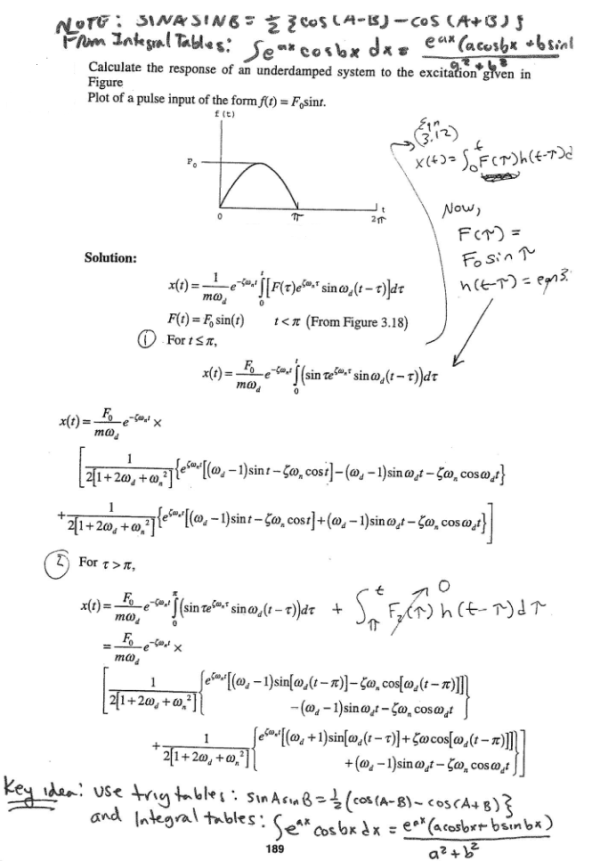Calculate the reponse of an underdamped system to the excitation given in the figure. Plot of a pules input of the form f(t)=F0sint.Calculate the reponse of an underdamped system to the excitation given in the figure. Plot of a pules input of the form f(t)=F0sint.

Vibrations Page 1 vibrations vibrations vibrations vibrations vibrations vibrations vibrations Vibrations Page 2